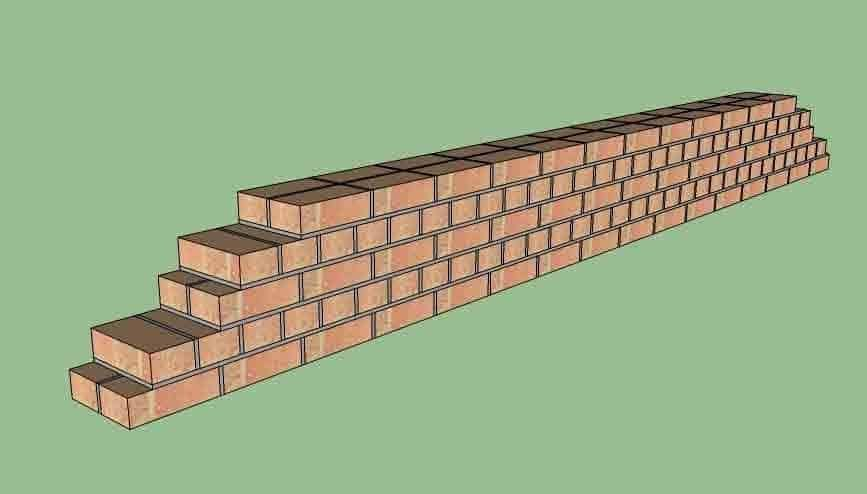# Calculation Of Bricks In A Wall

Contents

## Calculation Of Bricks In A Wall:

Estimation of building materials is essentially required before starting a new construction project. In our previous article, we have already discussed how to calculate cement, sand, and aggregates. Today we will discuss how to calculate number of bricks in a wall. This is a manual brick calculator that will help you to calculate no. of bricks for your project.

### Brick Calculator In Metric System:

For calculating the number of bricks, the thickness of plaster should be deducted from the thickness of the brick masonry wall. For example, for a standard brick wall (With load-bearing columns) of 9 inches or 230 mm thick, the actual thickness without plaster would be 200mm, i.e. one brick thick.To use the brick calculator manually we need some data for calculating no. of bricks.

Required Data:

1. Volume of the wall.

2. Volume of a standard brick.

3. Details of openings in the wall.

Let’s assume

Length of the wall = l = 4 m

Height of the wall = h = 3 m

Thickness of the wall = b = 200 mm = 0.2 m

Assuming that the wall has no openings.

Brick size = 190 mmx 90 mm x 90 mm (without mortar)

Volume of brick masonry in wall = lxhxb = 4 x 3 x 0.2 = 2.4 m3

Volume of one brick with mortar = 0.2 x 0.1 x 0.1 = 0.002 m3

Number of bricks = [Volume of brick masonry / volume of one brick]

= 2.4 / 0.002 = 1200 bricks.

Consider 5% wastages of bricks.

Thus, number of bricks required for 1 cubic meter = 500

#### Example 2 (Brick Calculator In Feet Unit):

You can use the same brick calculator for different units. In this example, We will use the feet unit.

1. First, calculate the volume of the wall to be built:

Let us assume,

The length of the wall (l) = 10 feet.

The height of the wall (h) = 10 feet.

Thickness of the wall (b) = 200 mm = 0.656 foot.

Calculate the volume of the wall by multiplying the length, height, and thickness.

Volume of the wall = l×h×b = 10×10×0.656 =65 Cu.F

2. Calculate the volume of one brick:

We will use standard Indian bricks for the calculator.

The standard size of a brick (IS Standard) is 190 mm ×90 mm ×90 mm and

with the mortar joints, it becomes 200mm × 100 mm× 100 mm.

l = 200 mm= 0.656168 ft.

b = 100 mm =0.328084 ft.

h = 100 mm = 0.328084 ft.

Volume of the brick = l× b×h =  0.656168× 0.328084× 0.328084 = 0.0706 Cu.F

3. To find out the total nos of brick divide the volume of the wall by the volume of the brick.

No. of bricks required = 65.6/0.0706 = 929 no. of bricks.

Note:

1. Consider 5% wastages of bricks.

2. If the wall has any openings such as doors, windows, etc subtract the volume of the openings from the volume of the wall and then divide it by the volume of brick.

I hope you have now learned how to calculate bricks using our manual brick calculator. Good luck.

Types Of Bricks Used In Building & Construction

How To Calculate No. Of Concrete Blocks In Wall

How To Calculate Concrete For Retaining Wall

Join Telegram Channel – Civil Engineering Daily

### 31 thoughts on “Calculation Of Bricks In A Wall”

• I’m waiting for that

• Thanks………….

1. Yaa it’s nice I like it…..me also a civil engineer student

2. NYC

3. Most easy technique

4. Why dont subtract mortar ?

5. Nyc, we can also use only areas

7. Very nice explanation
Which is easy to understanding..

• How to calculate quantity of material used in solid block work in 1m2?..

8. as we saw it is esay way to find number of bricks by this formula bt when we have to find nmbr of bricks for house ,where nmbr of walls is more here dificult to find it ..because its complex to find by single walls

9. Use ful information

10. Nice explain

11. Very nyc…….!! & easy for understanding properly.

12. So simple, easy and useful information.. Thks

13. Useful information

14. Use fully information…….03159410394…….

15. Please started with the whatsapp group helpful for civil engineering my number is 8248930422

16. Very nice……because esay to understanding and its thanks for giving useful information

18. Very very nice this is very important

thanks

20. How to calculate the rock toe of the bund